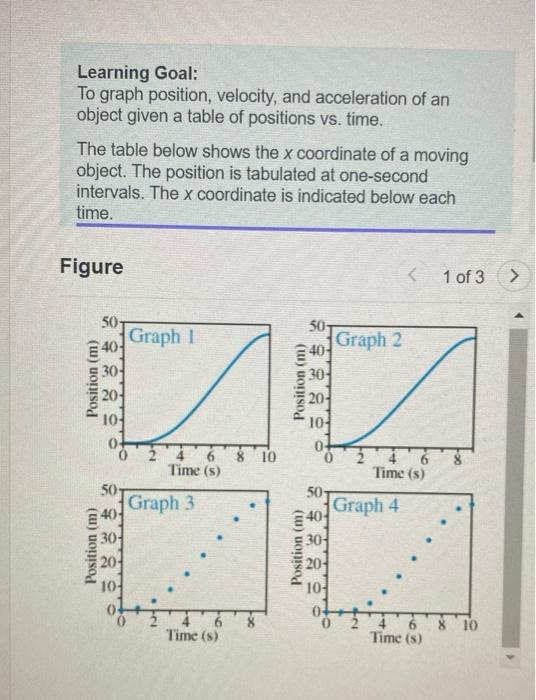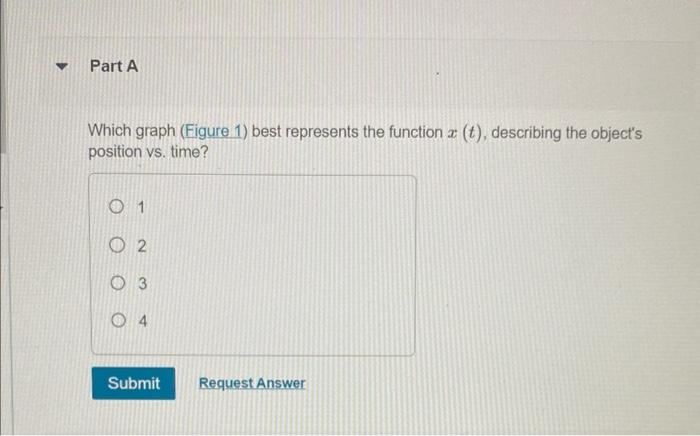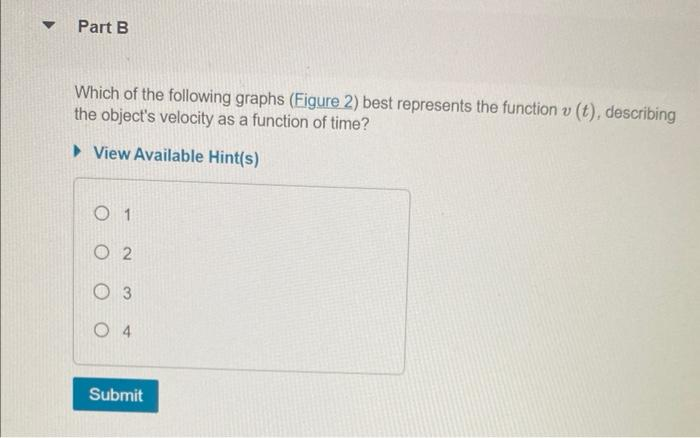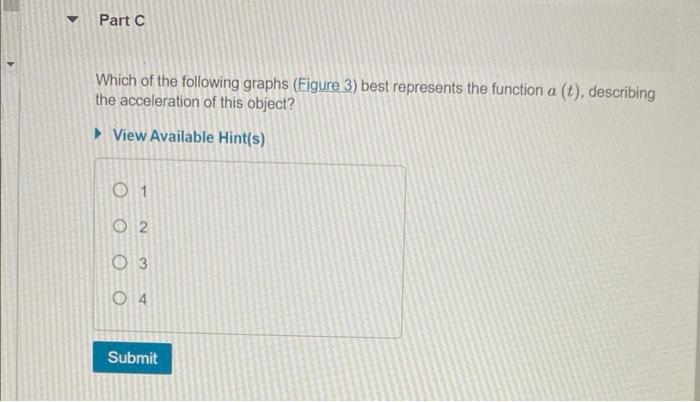# Question Solved1 AnswerLearning Goal: To graph position, velocity, and acceleration of an object given a table of positions vs. time. The table below shows the \( x \) coordinate of a moving object. The position is tabulated at one-second intervals. The \( x \) coordinate is indicated below each time. Figure 1 of 3 Which graph (Figure 1) best represents the function \( x(t) \), Learning Goal: To graph position, velocity, and acceleration of an object given a table of positions vs. time. The table below shows the \( x \) coordinate of a moving object. The position is tabulated at one-second intervals. The \( x \) coordinate is indicated below each time. Figure 1 of 3 Which graph (Figure 1) best represents the function \( x(t) \), describing the object's position vs. time? 1 5 3 4 Which of the following graphs (Figure 2 ) best represents the function \( v(t) \), describing the object's velocity as a function of time? View Available Hint(s) Which of the following graphs (Figure 3) best represents the function \( a(t) \), describing the acceleration of this object? View Available Hint(s)Transcribed Image Text: Learning Goal: To graph position, velocity, and acceleration of an object given a table of positions vs. time. The table below shows the \( x \) coordinate of a moving object. The position is tabulated at one-second intervals. The \( x \) coordinate is indicated below each time. Figure 1 of 3 Which graph (Figure 1) best represents the function \( x(t) \), describing the object's position vs. time? 1 5 3 4 Which of the following graphs (Figure 2 ) best represents the function \( v(t) \), describing the object's velocity as a function of time? View Available Hint(s) Which of the following graphs (Figure 3) best represents the function \( a(t) \), describing the acceleration of this object? View Available Hint(s)
More
Transcribed Image Text: Learning Goal: To graph position, velocity, and acceleration of an object given a table of positions vs. time. The table below shows the \( x \) coordinate of a moving object. The position is tabulated at one-second intervals. The \( x \) coordinate is indicated below each time. Figure 1 of 3 Which graph (Figure 1) best represents the function \( x(t) \), describing the object's position vs. time? 1 5 3 4 Which of the following graphs (Figure 2 ) best represents the function \( v(t) \), describing the object's velocity as a function of time? View Available Hint(s) Which of the following graphs (Figure 3) best represents the function \( a(t) \), describing the acceleration of this object? View Available Hint(s)